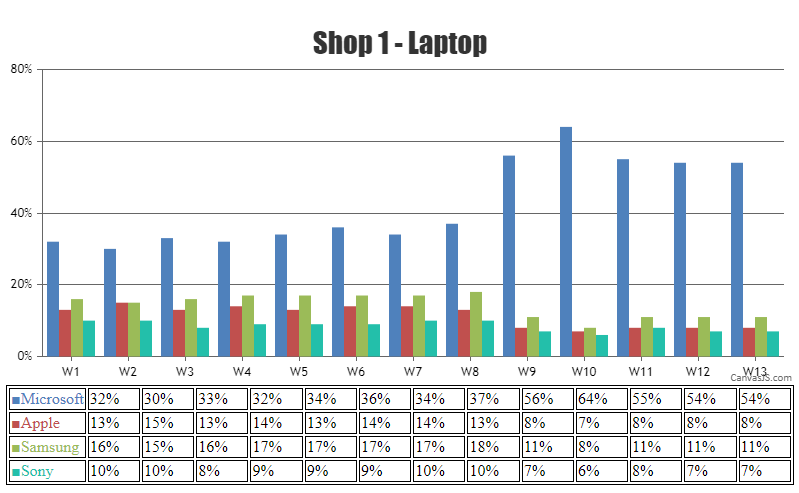Home Forums Chart Support Tabular presentation of graph data Reply To: Tabular presentation of graph data

#37571

It is not possible to create a graph in tabular form (based on your screenshot) using CanvasJS as of now. However, with a few lines of code, it is possible to create a table with data from the chart as shown in the code-snippet below,

``````function addTable(chart){
var tableData = "";
for(var i = 0; i < chart.data.length; i++){
tableData += "<tr>" + "<td style='color:" + chart.data[i].color + "'>■" + chart.data[i].name + "</td>";
for(var j = 0; j < chart.data[i].dataPoints.length; j++){
tableData += ("<td>" + chart.data[i].dataPoints[j].y +"%</td>")
}
tableData += "</tr>";
}
\$("#chartData").append(tableData)
}``````

Kindly take a look at this JSFiddle for an example on the same.